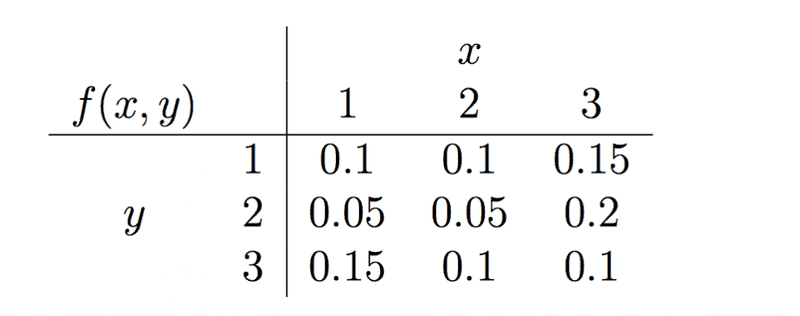# Joint probability distribution

## Homework Statement

4. Let X and Y have the joint probability distribution(a) Find P(X +Y ≤ 4).

(b) Find the marginal probability distributions f1(x) and f2(y).

(c) Find P(X < 2|Y = 2).

(d) Are X and Y independent?

## The Attempt at a Solution

a) f(1,1) + f(1,2) + f(1,3) + f(2,1) + f(2,2) + f(3,1)

b) f1(1) = .1 + .05 + .15 = .3
f1(2) = .1 + .05 + .1 = .25
f1(3) = .15 + .2 + .1 = .45

f2(1) = .35
f2(2) = .3
f2(3) = .35

c) f(1,2) = .05

d) they are independent if f(x,y) = f1(x)f2(y) for all x and y
f(1,1) = .1 f1(1)f2(1) = .3(.35) = .105
they are not independent .

is this correct?

andrewkirk
•